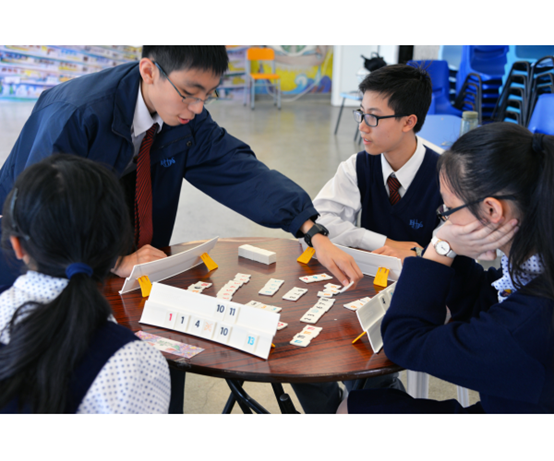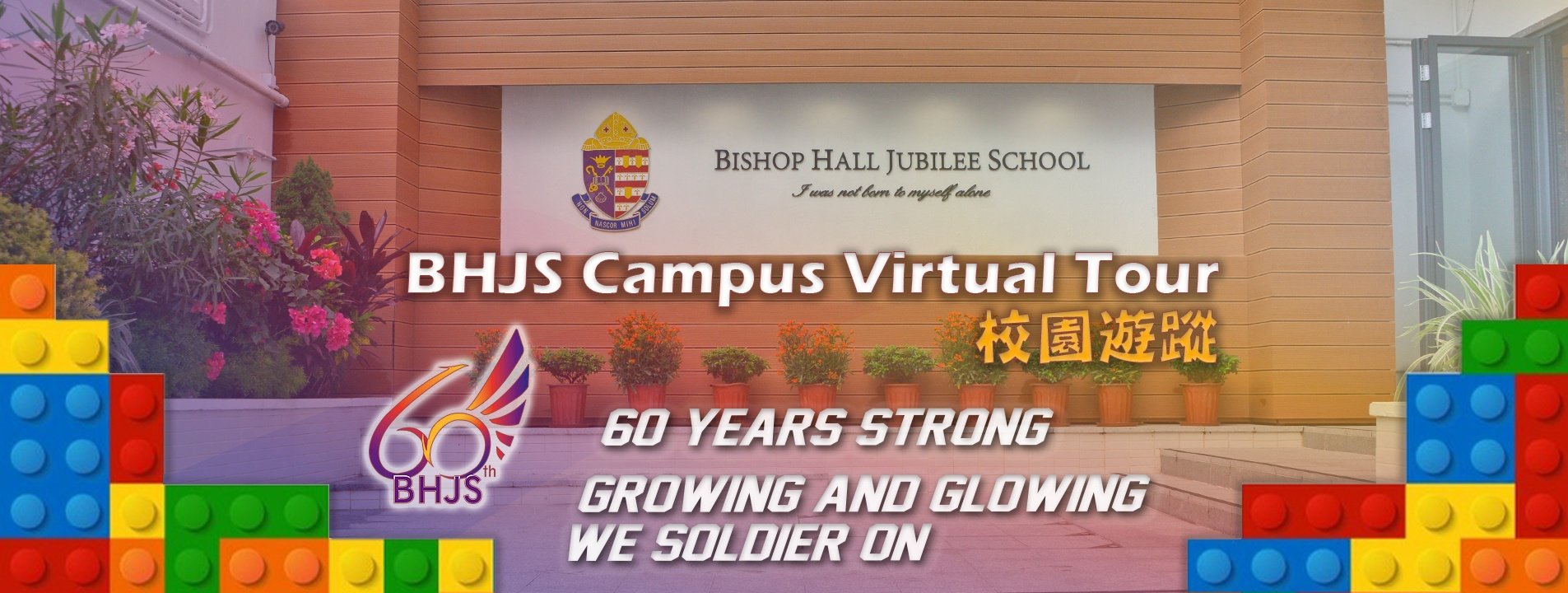60 YEARS STRONG
Growing and Glowing We Soldier OnSOLAR POWER PROJECT
Our Mission to a sustainable futureSERVICE LEARNING
We live yet we serve, We serve yet we learn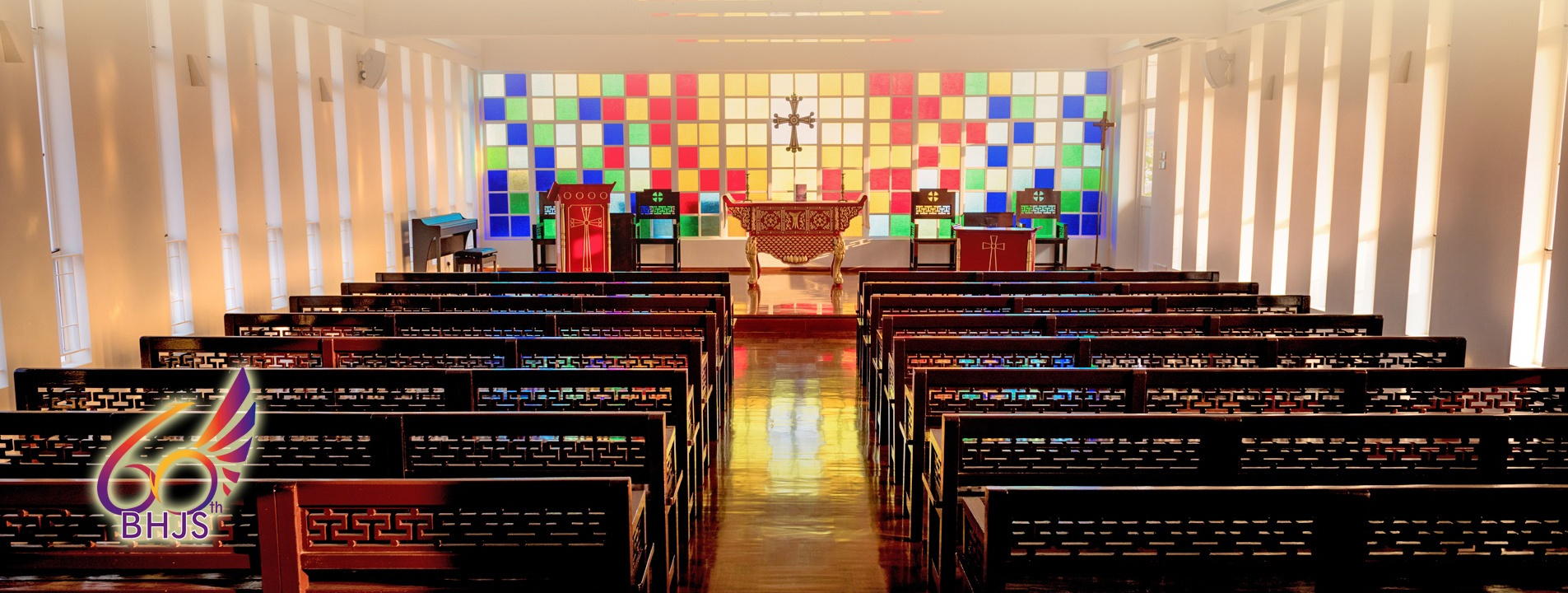CHRISTIAN EDUCATION
We rejoice in the knowledge of God's truth

Mathematics

Aims & Objectives

The overall curriculum aims of the Mathematics Education Key Learning Area are to develop in students:

• the ability to think critically and creatively, to conceptualise, inquire and reason mathematically, and to use mathematics to formulate and solve problems in daily life as well as in mathematical contexts and other disciplines;
• the ability to communicate with others and express their views clearly and logically in mathematical language;
• the ability to manipulate numbers, symbols and other mathematical objects;
• number sense, symbol sense, spatial sense, measurement sense and the capacity to appreciate structures and patterns;
• a positive attitude towards the learning of mathematics and an appreciation of the aesthetic nature and cultural aspects of mathematics.

Department Members
Department Head: Mr. Mak Chun Ho
Deputy Department Head: Mr. Chan Hung Kwan
Teachers: Mr. Au Wai Kay, Mr. Cheuk David Tai Wai, Ms. Chow Lok Yee, Mr. Lau Ho Long, Mr. Poon Wai Cheong Alex, Mr. Tang Wing Keung, Mr. Wong Siu Hang, Ms. Yip Ying Ha, Mr. Yu Yiu Tong

Curriculum (Junior Form)

Number and Algebra Strand
1. Basic computation
2. Directed numbers
3. Approximate values and numerical estimation
4. Rational and irrational numbers
5. Using percentages
6. Rates, ratios and proportions
7. Algebraic expressions
8. Linear equations in one unknown
9. Linear equations in two unknowns
10. Laws of integral indices
11. Polynomials
12. Identities
13. Formulae
14. Linear inequalities in one unknown

Measures, Shape and Space Strand
15. Errors in measurement
16. Arc lengths and areas of sectors
17. 3-D figures
18. Mensuration
19. Angles and parallel lines
20. Polygons
21. Congruent triangles
22. Similar triangles
24. Centres of triangles
25. Pythagoras’ theorem
26. Rectangular coordinate system
27. Trigonometry

Data Handling Strand
28. Organisation of data
29. Presentation of data
30. Measures of central tendency
31. Probability

Curriculum (Senior Form)
Number and Algebra Strand
1. Quadratic equations in one unknown
2. Functions and graphs
3. Exponential and logarithmic functions
6. Variations
7. Arithmetic and geometric sequences and their summations
8. Inequalities and linear programming
9. More about graphs of functions

Measures, Shape and Space Strand
10. Equations of straight lines
11. Basic properties of circles
12. Loci
13. Equations of circles

Data Handling Strand
15. Permutations and combinations
17. Measures of dispersion
18. Uses and abuses of statistics

Activities

Hang Lung Mathematics Awards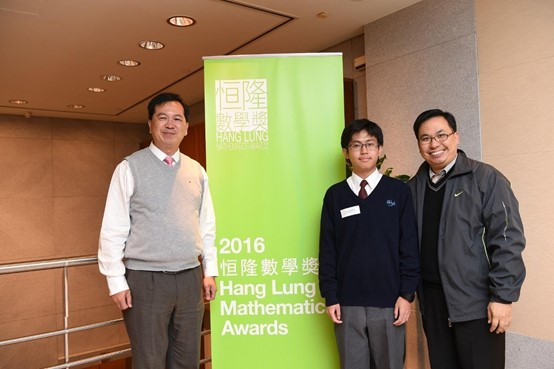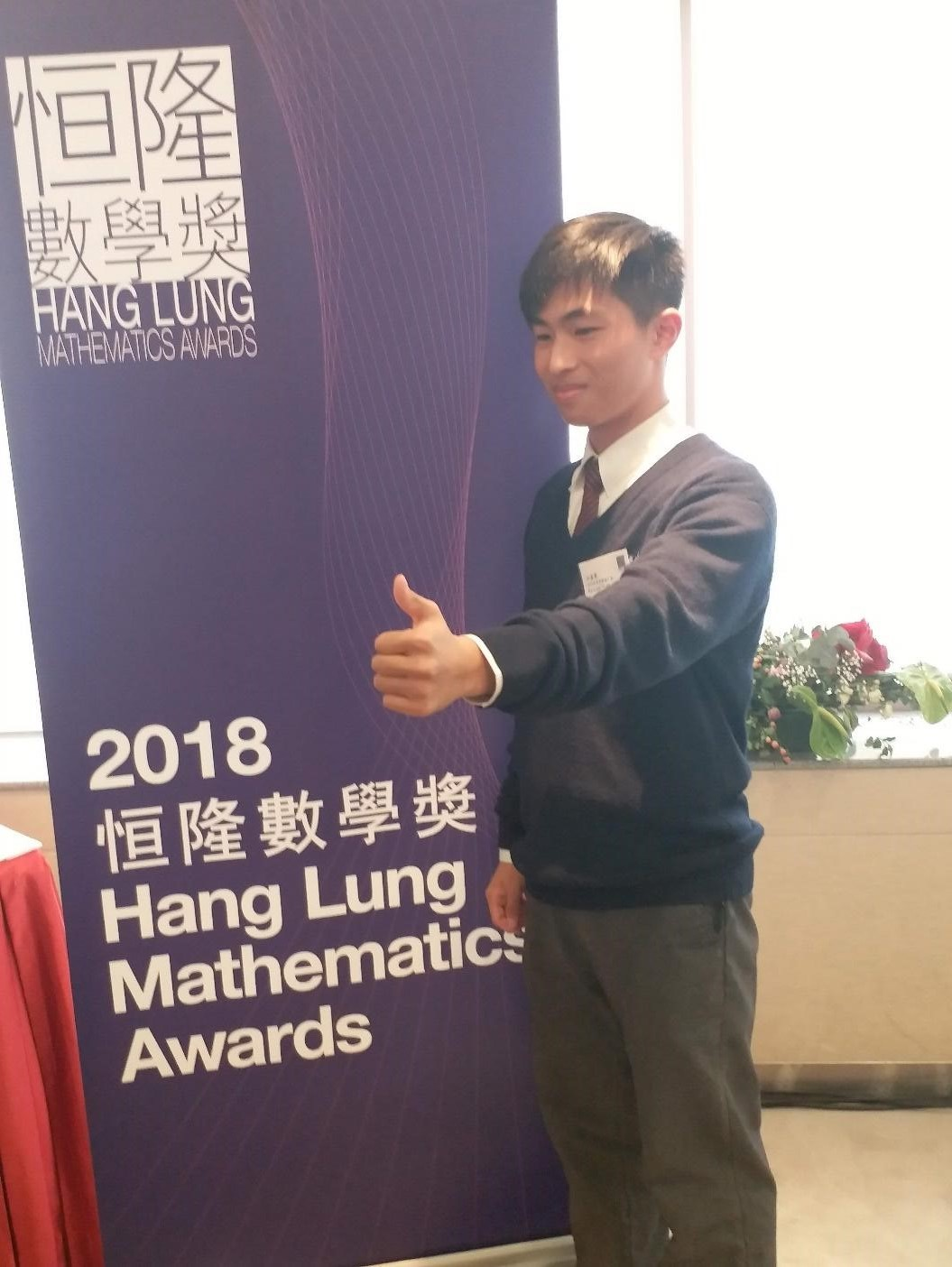Paper Tower Competition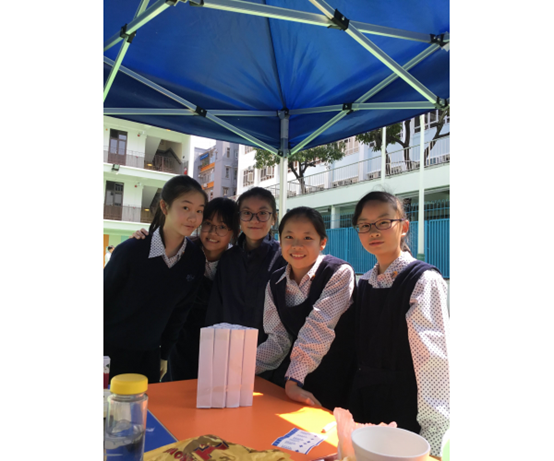Rummikub Competition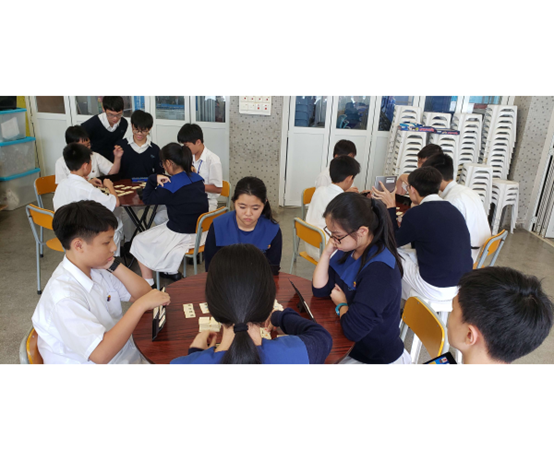Form 1 Magic 24 Competition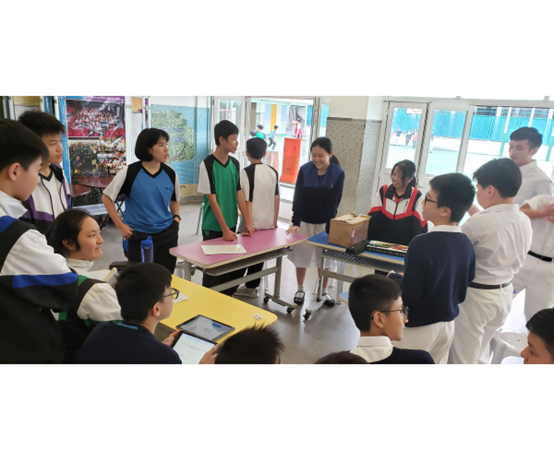STEM Week